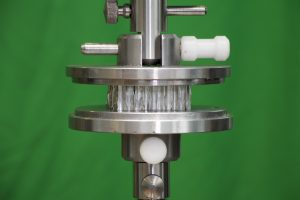## Identification of mechanical properties of composite materials and structures

The Institute has developed a numerical-experimental technique based on modal vibration testing for the determination of mechanical properties of composite materials. With the vibration-based approach, the elastic properties can be determined in a contactless way, which is a major advantage for small test samples or brittle materials. The general idea of mixed numerical-experimental technique is that instead of measuring the property of interest, indirect procedures measure a number of related quantities and derive the unknown property from the experimental values of these quantities. A numerical model is used to relate the physical property of interest to the measured quantities. Numerical models are usually formulated in such a way that they compute the response of a system using the applied inputs and system properties.  Then the inverse problem is used to derive a number of model parameters from the system’s response to a particular input. The inverse problem is solved by minimizing the difference between the numerical model response and the experimentally measured one. The method previously has been successfully applied for identification of mechanical properties of composite materials from the vibration tests as well as for determination of plastic properties of polymers from micro hardness tests.